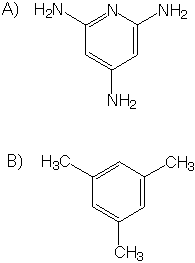Molecule Interactions Problem Set1) Which do you think has a higher boiling point, a neat (pure) solution of molecule A or a neat solution of molecule B?

2) Which do you think has a higher boiling point, a neat solution of molecule B or a neat solution of methane?

3) List the molecular interactions within neat liquids of A and of B. Give a one or two sentence description of each type of interaction.

4) What is the geometery of each atom of A?

5) What is the geometry of each atom of B?

6) Two water molecules a linked together by a hydrogen bond.

i) How far apart are the two oxygen atoms?
ii) How how long is the O-H covalent bond?
iii) How long is the O---H hydrogen bond?

7) Draw the tripeptide His-Arg-Lys. Label all sets of coplaner atoms. Give the orbital hybridization for each non-hydrogen atom.

8) Give the answers below in a diagram. Estimate free energy (deltaG), the enthalpy (deltaH), and the entropy contribution (TdeltaS) for transfer of one mole of each of the compounds A and B (above) from

i) vapor phase to neat liquid at 20 °C,
ii) neat liquid to dilute aqueous solution at 20 °C,
iii) vapor phase to neat liquid at 150 °C, and
iv) neat liquid to aqueous solution at 150 °C.